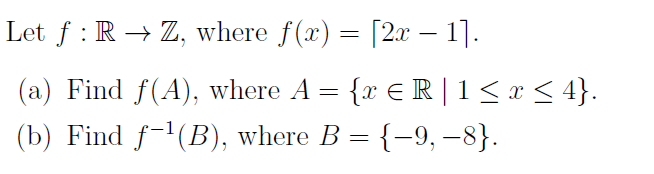# Let f : R → Z, where f(x) = [2x – 1].(a) Find f(A), where A = {x € R | 1< x < 4}.(b) Find f-'(B), where B = {-9, –8}.

Question
11 views

thank youhelp_outlineImage TranscriptioncloseLet f : R → Z, where f(x) = [2x – 1]. (a) Find f(A), where A = {x € R | 1< x < 4}. (b) Find f-'(B), where B = {-9, –8}. fullscreen
check_circle

star
star
star
star
star
1 Rating
Step 1

From the given s...

### Want to see the full answer?

See Solution

#### Want to see this answer and more?

Solutions are written by subject experts who are available 24/7. Questions are typically answered within 1 hour.*

See Solution
*Response times may vary by subject and question.
Tagged in

### Math